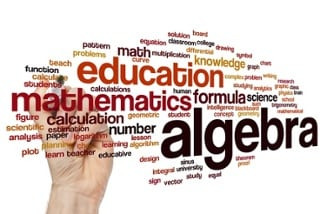Select Page## 4 Misconceptions Students Have About Multiplication

Although it is the third mathematical operation that students are exposed to, multiplication tends to be more challenging to students than their previous encounters with addition and subtraction. Yes, a student may be able to memorize their multiplication facts with...## 4 Common Misconceptions Students Have About Algebra

In the United States, algebra is seen as the gateway course to higher-level math courses and, in some cases, even college. This is oftentimes a student’s first encounter with abstract mathematical thinking, which leads to confusion and uncertainty. Here are four...## Demystifying the Common Core Standards for Mathematical Practice

Today, according to a school district’s curriculum, you have two standards to teach. The first is a content standard: “Find the roots, real and imaginary, of a quadratic equation.” The second is one of the Common Core Standards for Mathematical...